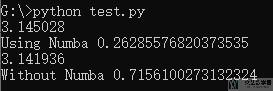# Python性能优化

• A+

## 性能优化

### numba

1. import time
2. import random
3. from numba import jit, autojit, int32
4. def no_monte_carlo_pi(nsamples):
5.     acc = 0
6.     for i in range(nsamples):
7.         x = random.random()
8.         y = random.random()
9.         if (x ** 2 + y ** 2) < 1.0:
10.             acc += 1
11.     return 4.0 * acc / nsamples
12. @jit(nopython=True)
13. def monte_carlo_pi(nsamples):
14.     acc = 0
15.     for i in range(nsamples):
16.         x = random.random()
17.         y = random.random()
18.         if (x ** 2 + y ** 2) < 1.0:
19.             acc += 1
20.     return 4.0 * acc / nsamples
21. if __name__ == '__main__':
22.     t1 = time.time()
23.     print(monte_carlo_pi(1000000))
24.     t2 = time.time()
25.     print('Using Numba',t2-t1)
26.     # ------Without Numba
27.     t1 = time.time()
28.     print(no_monte_carlo_pi(1000000))
29.     t2 = time.time()
30.     print('Without Numba',t2-t1)numba文档 : https://numba.pydata.org/

## 其他

• 微信公众号
• 关注微信公众号
•• QQ群
• 我们的QQ群号
•